# Tate algebra

(Redirected from Affinoid algebra)
Jump to: navigation, search

Letbe a field which is complete with respect to an ultrametric valuation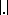(i.e.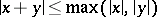). The valuation ringhas a unique maximal ideal,. The field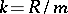is called the residue field of.

Examples of such fields are the local fields, i.e. finite extensions of the-adic number field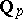, or the field of Laurent series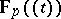inwith coefficients in the finite field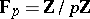(cf. also Local field).

Let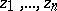denote indeterminates. Then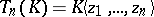denotes the algebra of all power series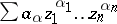with() such that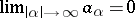(). The norm on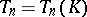is given by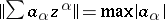. The ring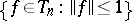is denoted by, andis an ideal of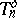. Thenis easily seen to be the ring of polynomials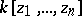.

The-algebrais called the free Tate algebra. An affinoid algebra, or Tate algebra,overis a finite extension of some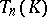(i.e. there is a homomorphism of-algebras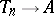which makesinto a finitely-generated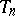-module). The space of all maximal ideals,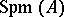of a Tate algebrais called an affinoid space.

A rigid analytic space overis obtained by glueing affinoid spaces. Every algebraic variety overhas a unique structure as a rigid analytic space. Rigid analytic spaces and affinoid algebras were introduced by J. Tate in order to study degenerations of curves and Abelian varieties over.

The theory of formal schemes over(the valuation ring of) is close to that of rigid analytic spaces. This can be seen as follows.

Fix an elementwith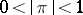. The completion ofwith respect to the topology given by the ideals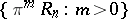is the ring of strict power series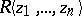over. Now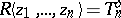, andis the localization ofwith respect to. So one can viewas the "general fibre" of the formal schemeover. More generally, any formal schemeovergives rise to a rigid analytic space over, the "general fibre" of. Non-isomorphic formal schemes overcan have the same associated rigid analytic space over. Further, any reasonable rigid analytic space overis associated to some formal scheme over.

Affinoid spaces and affinoid algebras have many properties in common with affine spaces and affine rings over. Some of the most important are: Weierstrass preparation and division holds for(cf. also Weierstrass theorem); affinoid algebras are Noetherian rings, and even excellent rings if the fieldis perfect; for any maximal idealof an affinoid algebrathe quotient field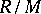is a finite extension of; many finiteness theorems; any coherent sheafon an affinoid spaceis associated to a finitely-generated-module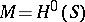(further: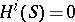for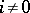).

Another interpretation ofis:consists of all "holomorphic functions" on the polydisc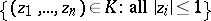. This interpretation is useful for finding the holomorphic functions on more complicated spaces like Drinfel'd's symmetric spaces. Letbe a local field with algebraic closure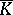. Then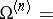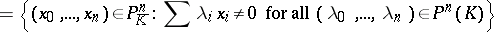is a Drinfel'd symmetric space.

Spaces of this type have been used for the construction of Tate's elliptic curves (cf. Tate curve), Mumford curves and surfaces, Shimura curves and varieties, etc.